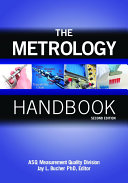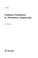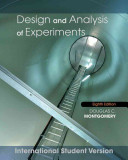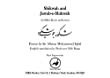### Measurement Theory

Data is displayed for academic year: 2022./2023.

#### Course Description

Data Measurement. Fields: monitoring, research, development, measurement, control. DMS (Data Measurement System): definition, structure, outer and inner limits, classification according to the information and energy.Experiment. Fundamentals and terms (requirements, limits, repeatability). Carrying out of an experiment and records. Error analysis. Direct measurements and weighted factors. Quantities measured indirectly. Weighted regression functions, uncertainty of parameters. Application of matrix in the error analysis. Uncertainty of the predicted value. Conditional measurements and conditional equations. Calculation of corrections. Examples. Mathematical model of measurand. Parameters of real measurements (final resolution, hysteresis, arithmetic of final precision, non-corrected values). Correlated and non-correlated quantities. Effective level of freedom and level of confidence. Examples.Results of measurement. Rejection of result according to Chauvenet criterion.

#### Study Programmes

[FER3-EN] Control Systems and Robotics - profile
Elective course (3. semester)
Elective courses (1. semester)
[FER3-EN] Data Science - profile
Elective courses (1. semester)
Recommended elective courses (3. semester)
[FER3-EN] Electrical Power Engineering - profile
Elective courses (1. semester) (3. semester)

#### Learning Outcomes

1. Recall diferent contributions to the uncertainty of measurement
2. Estimate uncertainty of measurement for uncorrelated quantities
3. Employ Gauss distribution for determination of expanded uncertainty
4. Analyze indirect and conditional measurements
5. Create self-developed algorithms for regression functions
6. Assess different approaches in the estimation of measurement results

#### Forms of Teaching

Lectures

Using the modern methods

Exercises

Solving the examples of calculations

Independent assignments

Homework

Laboratory

Example from the laboratory praxis

#### Week by Week Schedule

1. Design of experiment, Definition of metrology terms
2. Least-squares theory
3. Direct measurements and weighted factors
4. Uncertainty of measurement
5. Indirectly measured quantities
6. Correlated and uncorrelated input quantities
7. Coverage factor and effective degree of freedom
8. Midterm exam
9. Use of matrixes in the error analysis
10. Weighted regression functions and uncertainty of the expected value
11. Linearization of non-linear functions
12. Conditional equations and measurements
13. Criterion for rejection of measurement results
14. Monte Carlo methods
15. Final exam

#### Literature

JCGM 200:2012 (2012.), International vocabulary of basic and general terms in metrology (VIM), 3rd edition, BIPM
JCGM 100:2008 (2008.), Evaluation of measurement data – Guide to the expression of uncertainty in measurement (GUM), BIPM
BIPM (2019.), The International System of Units (SI), 9th edition, BIPMJ. Bucher (2012.), The Metrology Handbook, ASQM.J.Allen, W.M. Yen (2002.), Introduction to Measurement Theory, Waveland Press, Inc.S.G. Rabinovich (20056.), Measurement Errors and Uncertainties, Springer
J.R. Taylor (1997.), An introduction to error analysis, University Science BooksD.C. Montgomery (2012.), Design and Analysis of Experiments (8th Edition), WileyB.E. Cooper (2014.), Statistics for experimentalists, Pergamon Press
V. Bego (2003.), Mjerenja u elektrotehnici, Graphis, Zagreb
N. Čubranić (1967.), Teorija pogrešaka s računom izjednačenja, Tehnička knjiga, Zagreb

#### General

ID 223690
Winter semester
5 ECTS
L3 English Level
L1 e-Learning
30 Lectures
0 Seminar
6 Exercises
13 Laboratory exercises
0 Project laboratory